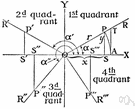# trigonometry

(redirected from Trigonometric Ratios)
Also found in: Thesaurus, Encyclopedia.
Related to Trigonometric Ratios: Trigonometric functions

## trig·o·nom·e·try

(trĭg′ə-nŏm′ĭ-trē)
n.
The branch of mathematics that deals with the relationships between the sides and the angles of triangles and the calculations based on them, particularly the trigonometric functions.

[New Latin trigōnometria : Greek trigōnon, triangle; see trigon + Greek -metriā, -metry.]

## trigonometry

(ˌtrɪɡəˈnɒmɪtrɪ)
n
(Mathematics) the branch of mathematics concerned with the properties of trigonometric functions and their application to the determination of the angles and sides of triangles. Used in surveying, navigation, etc. Abbreviation: trig
[C17: from New Latin trigōnometria from Greek trigōnon triangle]

## trig•o•nom•e•try

(ˌtrɪg əˈnɒm ɪ tri)

n.
the branch of mathematics that deals with the relations between the sides and angles of plane or spherical triangles, and the calculations based on them.
[1605–15; < New Latin trigōnometria; see trigon, -o-, -metry]

## trig·o·nom·e·try

(trĭg′ə-nŏm′ĭ-trē)
The study of the properties and uses of trigonometric functions.

## trigonometry

the branch of mathematics that treats the measurement of and relationships between the sides and angles of plane triangles and the solid figures derived from them. — trigonometric, trigonometrical, adj.
ThesaurusAntonymsRelated WordsSynonymsLegend:
 Noun 1trigonometry - the mathematics of triangles and trigonometric functionstrigmath, mathematics, maths - a science (or group of related sciences) dealing with the logic of quantity and shape and arrangementpure mathematics - the branches of mathematics that study and develop the principles of mathematics for their own sake rather than for their immediate usefulnessspherical trigonometry - (mathematics) the trigonometry of spherical trianglestriangulation - a trigonometric method of determining the position of a fixed point from the angles to it from two fixed points a known distance apart; useful in navigation
Translations
trigonometrie
trigonometria
trigonometri

## trigonometry

[ˌtrɪgəˈnɒmɪtrɪ] N

## trigonometry

[ˌtrɪgəˈnɒmətri] n

## trigonometry

[ˌtrɪgəˈnɒmɪtrɪ] ntrigonometria
References in periodicals archive ?
11.2###(ii) Define trigonometric ratios of an acute angle.
Yes, I am depriving my daughter of all the pleasures of a traditional schooling and I have taken on the responsibility of teaching trigonometric ratios and electromagnetic forces, but I followed my heart and not my head and broke all the rules by not conforming to the traditional.
In Bhattacharjee (2012), the discovery of flaw in the traditional definitions of trigonometric ratios, which make use of the most unrealistic concept of negative length or distance has been reported.
G-SRT.8 Use trigonometric ratios and the Pythagorean Theorem to solve right triangles in applied problems.
38-45) and trigonometric ratios are formally introduced in Year 9 when students "use similarity to investigate the constancy of the sine, cosine and tangent ratios for a given angle in right-angled triangles" (ACARA, 2010, p.
Mathematics in the final year of middle school includes: Square Roots, Irrational Numbers, Factorization of Polynomials, Quadratic Equations, Graphs of Quadratic Functions, Pythagorean Theorem, Geometry of Circles, Trigonometric Ratios, Correlation.
Comfortable with trigonometric ratios (Cosiness/Cosines)
For example, demonstrating the trigonometric ratios using The Geometer's Sketchpad (Jackiw 1995) is not as effective as using the software to explore, model, and subsequently verify the functions.
This paper reports on the discovery of flaws in the traditional definitions of trigonometric ratios (Das & Mukherjee, 1999; Loney, 1947; Hall & Knight, 1949; Briggs & Bryan, 1950; Carslaw, 1948; Nag & Basu, 1997; De, 1998; Blackburn, 1871; Sy, 2008; Maor, 2002; Lock, 1896; Paterson, 1911) of an angle, which (in most cases) make use of the most unrealistic concept of negative length (or distance).
We asked the students how one can prove the lemma and they gave suggestions such as finding the heights of the two triangles, and considering trigonometric ratios. With minimal help, one student volunteered to present his proof to the rest of the class as follows:
Problems involving the use of trigonometric ratios are closely related to rate problems.
Those introduced to the subject via triangle trigonometry, which is more comprehensible than circle trigonometry at the early stage of learning (Kendal & Stacey 1997), have (i) to relate triangular pictures to numerical relationships, (ii) to cope with trigonometric ratios, and (iii) to manipulate the symbols involved in such relationships (Blackett & Tall, 1991).

Site: Follow: Share:
Open / Close